# Basic notions and properties of functions

Function. Domain and codomain of a function.
Rule (law) of correspondence. Monotone function.
Bounded and unbounded function. Continuous and
discontinuous function. Even and odd function.
Periodic function. Period of a function.
Zeros (roots) of a function. Asymptote.

Domain and codomain of function. In elementary mathematics we study functions only in a set of real numbers R . This means that an argument of a function can adopt only those real values, at which a function is defined, i.e. it also adopts only real values. A set X of all admissible real values of an argument x , at which a function y = f ( x ) is defined, is called a domain of a function . A set Y of all real values y , that a function adopts, is called a codomain of a function . Now we can formulate a definition of a function more exactly: such a rule (law) of a correspondence between a set X and a set Y , that for each element of a set X one and only one element of a set Y can be found, is called a function . From this definition it follows, that a function is given if :
- the domain of a function X is given;
- the codomain of a function Y is given;
- the correspondence rule ( law ), is known.
A correspondence rule must be such, that for each value of an argument only one value of a function can be found. This requirement of a single-valued function is obligatory.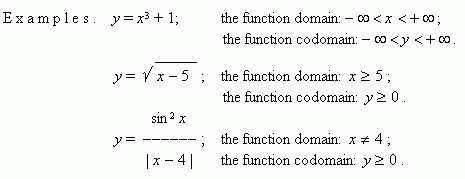Monotone function. If  for any two values of an argument x 1 and x 2 from the condition x 2 > x 1 it follows f ( x 2 ) > f ( x 1 ), then a function is called increasing ; if for any x 1 and x 2 from the condition x 2 > x 1 it follows f ( x 2 ) < f ( x 1 ), then a function is called decreasing .A function, which only increases or only decreases, is called a monotone function.

Bounded and unbounded functions. A function is bounded , if such positive number M exists, that | f ( x ) |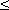M for all values of x . If such positive number does not exist, then this function is unbounded .

E x a m p l e s.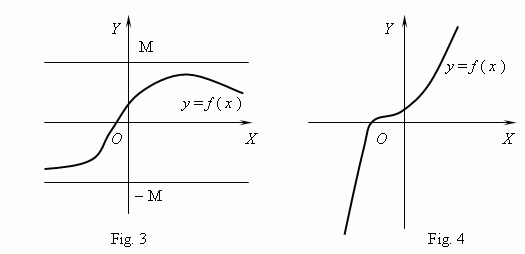A function, shown on Fig.3, is a bounded, but not monotone function. On Fig.4 quite the opposite, we see a monotone, but unbounded function. ( Explain this, please ! ).

Continuous and discontinuous functions. A function y = f ( x ) is called a continuous function at a point x = a, if:
1)  the function is defined at x = a , i.e. f ( a ) exists;
2)  a finite lim f ( x )  exists;
x a
( see the paragraph "Limits of functions" in the section “Principles of analysis”)

3) f ( a ) = lim f ( x ) .
x a

If even one from these conditions isn’t executed, this function is called discontinuous at the point x = a .

If a function is continuous at all points of its domain, it is called a continuous function .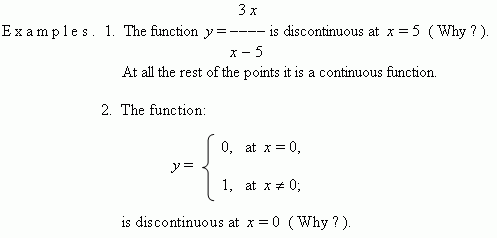Even and odd functions. If for any x from a function domain: f ( – x ) = f ( x ), then this function is called even ;
if f ( – x ) = – f ( x ), then this function is called odd . A graph of an even function is symmetrical relatively y -axis ( Fig.5 ), a graph of an odd function is symmetrical relatively the origin of coordinates ( Fig.6 ).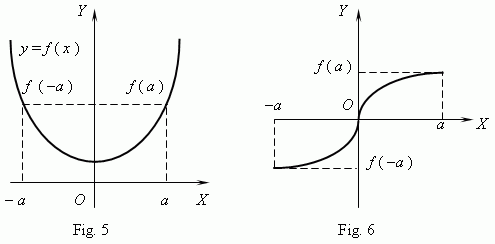Periodic function. A function f ( x ) is periodic , if such non-zero number T existsthat for any x from a function domain:
f ( x + T ) = f ( x ). The least such number is called a period of a function . All trigonometric functions are periodic.

E x a m p l e   1 .   Prove that  sin x has a number 2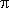as a period.

S o l u t i o n .       We  know, that  sin ( x+ 2n ) = sin x ,  where n = 0, ± 1, ± 2, …
Hence, adding  2n to an argument of a sine doesn’t change its value.
Maybe another number with the such property exists ?
Assume, that P is the such number, i.e. the equality:

sin ( x + P ) = sin x ,

is valid for any value of x . Then this is valid for x =/ 2 , i.e.

sin (/ 2 + P ) = sin/ 2 = 1.

But  sin (/ 2 + P ) = cos P according to the reduction formula.Then from
the two last expressions it follows, that cos P = 1, but we know, that this
equality is right only if P = 2n . Because the least non-zero number of
2n is 2, this is a period of  sin x . It is proved analogously, that 2is also
a period for  cos x .
Prove, please, that functions tan x and cot x haveas a period.

E x a m p l e   2.    What number is a period for the function sin 2 x ?

S o l u t i o n .        Consider

sin 2 x = sin ( 2 x + 2n ) = sin [ 2 ( x +n ) ].

We see, that addingn to an argument x , doesn’t change the function value.
The least non-zero number ofn is, so this is a period of sin 2 x .

Zeros of function. An argument value, at which a function is equal to zero, is called a zero ( root ) of the function. It can be that a function has some zeros. For instance, the function y = x ( x + 1 ) ( x – 3)  has the three zeros: x = 0, x = – 1, x = 3 . Geometrically, a zero of a function is x -coordinate of a point of intersection of the function graph and x -axis. On Fig.7 a graph of a function with zeros x = a , x = b and x = c is represented.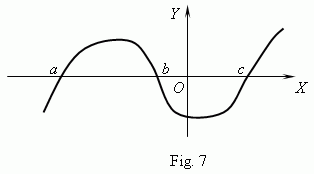Asymptote. If a graph of a function unboundedly approaches to some straight line at itstaking off an origin of coordinates, then this straight line is called an asymptote .